A Fresh Exploration of Relativity Theory

by

David Bryan Wallace

[This paper is a reproduction of my earliest essay on relativity theory, written in 1997. The digital original was lost, but a recently found print-out enabled me to re-create it, with a few typo corrections and clarity improvements.]

Certain customary features of twentieth century relativity are not required by the physical theory but are simply precedent following conventions. Moreover, these conventions frequently obscured the physics and led to error. Some of the errors gained greater popular acceptance than correct theory. Here presented is a careful derivation of relativity without the troublesome conventions.

Section 1: Implications of Michelson and Morley's observations.

Let us first, without introducing any new concepts, recapitulate the calculation of Michelson and Morley that guided their expectation. Remember that Michelson and Morley assumed dimensional invariance, i. e. neither length contraction nor time dilation.

Figure 1 represents the passage, at light-speed c relative to the fixed points A, B, C, of a signal from the point A to the point B and thence, being reflected, to point C; and the passage of an observer moving inertially at velocity v through point A at the moment of the origin of the signal there, to the point C at the moment when the signal reaches C. Point D is the fixed point through which the moving observer passes simultaneous in the fixed system with the reflection of the light signal at point BThe angle between the interferometer's velocity v and the interferometer's orientation is labeled φ. Let us derive a generalized equation for φ. Then, we can write the two special cases that Michelson and Morley mentioned, φ = 0° and φ = 90°.

The cosine law and quadratic formula yield solutions for t1 and t2 in the triangles ADB and CDB respectively. First, t1 from triangle ADB in equations (1) through (3):(1) cosine law for ΔADB(2) eq1 in standard quadratic form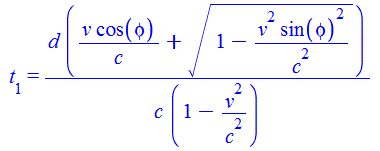(3) quadratic formula solution

Then, t2 from triangle CDB in equations (4) through (6):(4) cosine law for ΔCDB(5) eq4 in standard quadratic form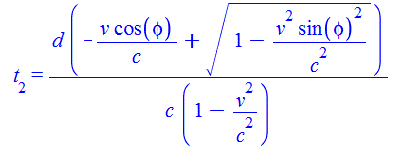(6) quadratic formula solution

If the moving observer agreed with the fixed observer's measurements of time and distance as Michelson and Morley expected, then the moving observer would observe the speed of light relative to himself to be twice the distance d divided by the total time as in equation (7):(7) hypothetical apparent light speed

However, the empirical outcome of the Michelson Morley experiment suggests that the moving observer will measure the speed of light relative to himself as c, not as indicated in equation (7). Therefore the moving observer does not agree with the fixed observer's measurements of time and distance. Before launching into speculation to explain this unexpected outcome, note that the experiment of Michelson and Morley did not utilize any clocks or time measurement, nor did it involve any notions of synchronization at a distance. It yields evidence regarding interferometric measurement of length, but nothing about measurement of time. The measurement of length by a solid measuring stick apparently yields identical measures as the measurement of length by interferometry, independent of motion. This, of course, must be true for the interferometric standardization of length measure to work as practiced by national and international standards organizations.

The reason interferometric length and measuring stick length did not differ as expected by Michelson and Morley was rightly conjectured by FitzGerald: "... the length of material bodies changes, according as they are moving through the ether or across it, by an amount depending on the square of the ratio of their velocity to that of light." If the electromagnetic forces that constitute atomic bonds within solid matter, which give it its solid qualities including dimensional stability, so act as to keep length dimensions stable as determined by interferometry, is it not then obvious that the physics of the bond is interferometer-like or, equivalently, a standing wave resonance. Bonds being electromagnetic in nature, it would be odd were it otherwise. This is sufficient to account for Michelson and Morley's observations. This postulate is the cornerstone of this exploration into relativity theory. Unlike FitzGerald, Lorentz (whose precedent we shall not follow) assumed invariance of dimensions perpendicular to the velocity.

Section 2: Transformation of lengths and angles from the fixed viewpoint.

In this exploration of relativity we do not follow Lorentz's precedent; we devise transformations to use instead of Lorentz transformations. Based on the empirical result of Michelson and Morley, we assume that moving observers will find the round trip speed of light in their frame of reference to be c because they rely on their local measuring sticks or interferometers to standardize their metrics.

Because it is specified that light moves at speed c relative to our fixed points, we define simultaneity in the fixed system as proposed by Einstein, by the process of reflecting light signals between pairs of points with the stipulation that time required for transmission is the same in each direction. Note that we shall not use this procedure to define simultaneity in a moving system, but shall make the fixed system the standard for time measurements and synchronization determinations, thus we conform to the SI standard of time based on a cesium atom at rest. We shall not assume that clocks are immune to rate changes due to motion, but we shall not say that the clock's rate change is a change in the rate of time. The moving observer will need to know his absolute velocity and the transformations between his local frame and the rest frame. His contracted measuring stick will yield a larger length measure than the stationary measure. [The subscript β indicates the moving observer's value.]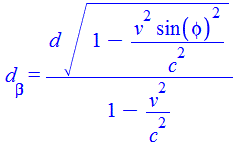(8) length transformation

When φ=π/2, sin(φ)=1, we have the relativistic transformation for lengths and velocities perpendicular to the relative motion.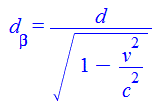(9) perpendicular length transformation

When φ=0, sin(φ)=0, we have the relativistic transformation for lengths and velocities parallel to the relative motion.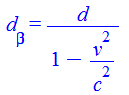(10) parallel length transformation

Therefore, the relativistic transformation for the relative motion itself cannot be other than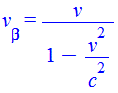(11) relative velocity transformation

Because the tangent of the angle φ is a perpendicular length divided by a parallel length the transformation for tan(φ) is(12) transformation of tan(φ)

Similarly, the sine of φ is a perpendicular length divided by a length at angle φ, so the transformation of sin(φ) is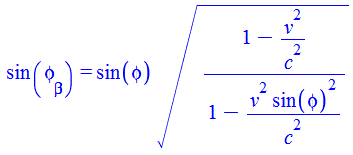(13) transformation of sin(φ)

Section 3: Introducing two convenient changes of variables.

Equation 11 can be written in standard quadratic form:(14)

Two changes of variables will facilitate derivation of inverses. Let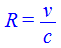(15)

and let(16)

Then equation (14) becomes(17)(18)

Also from equation (17) are other useful identities,(19)(20)(21)(22)

Now we can easily represent R and B in either frame of reference:(23)(24)

and their positive integral powers:(25)(26)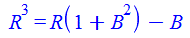(27)(28)(29)(30)

and so on.

Section 4: Inverse transformation for relative velocity and for parallel and perpendicular length.

Each of the relationships in section 2 has an inverse that is the transformation of the moving observer's measurement to the fixed viewpoint. From equation (15) we have:(31)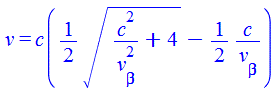(32)

Similarly, the inverse for length when φ = 0 [from equations (10) and (20)]:(33)

Solve for d and substitute for B and R [from equations (23) and (24)]:(34)

This is the inverse for length parallel to the velocity of the moving observer, φ = 0.

For the perpendicular case, when φ = π/2:(35)

Solve for d and substitute for B and R [from equations (23) and (24)]: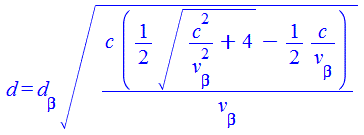(36)

This is the inverse for length perpendicular to the velocityof the moving observer, φ = π/2.

Section 5: Inverse transformation for angles.

Beginning with the relationship for tangent established in section 2:(37)

Solve for tan(#phi;) and substitute for B and R [from equations (23) and (24)]:(38)

For sine, square both sides of the relationship established in equation (13) and substituting 1-BR for R2 from equation (19)(39)

Solve for sin2(φ).(40)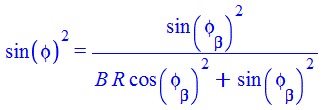(41)

Take the square root of each side and substitute for B and R [from equations (23) and (24)]: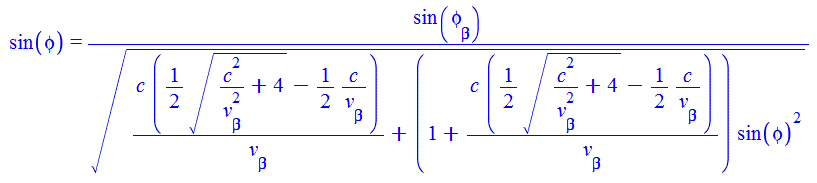(42)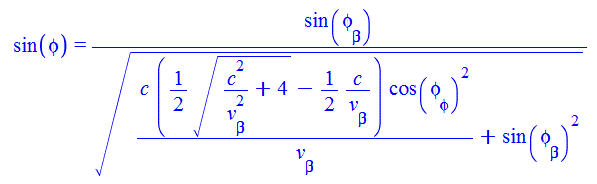(43)

Section 6: Inverse transformation for length in general.

From equation (8)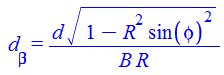(44)

Solve for d and substitute for sin2(φ) from equation (40)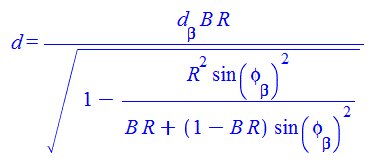(45)(46)(47)(48)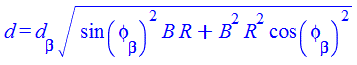(49)

Substitute for B and R from equations (23), (24), (25) and (26)(50)

Section 7: Table of example values

The maximum difference between φ and φβ occurs when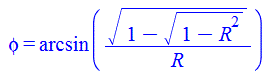(51)

 R φ in degrees where max φ-φβ occurs0.999 78.060794 499.74987 0.998 75.887004 249.74975 0.996 73.35737 124.7495 0.992 70.43989 62.248996 0.984 67.115389 30.997984 0.968 63.391453 15.370935 0.936 59.319519 7.5542355 0.872 55.021558 3.639156 0.744 50.736662 1.6664278 0.618034 48.438143 1 0.5 47.058597 0.6666667 0.25 45.462203 0.2666667 0.125 45.112789 0.1269841 0.0625 45.028031 0.0627451 0.03125 45.006998 0.0312805 0.015625 45.001749 0.0156288